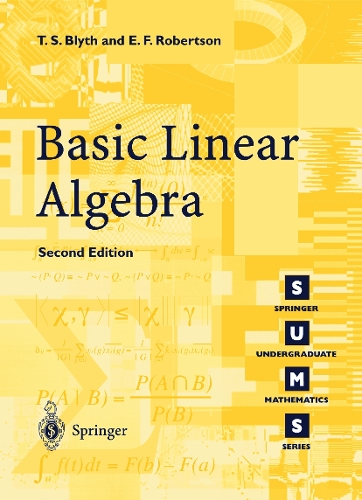•# Basic Linear Algebra - Springer Undergraduate Mathematics Series (Paperback)

(author), (author)
£24.95
Paperback 232 Pages / Published: 26/06/2002
• In stock

Available

Basic Linear Algebra is a text for first year students leading from concrete examples to abstract theorems, via tutorial-type exercises. More exercises (of the kind a student may expect in examination papers) are grouped at the end of each section. The book covers the most important basics of any first course on linear algebra, explaining the algebra of matrices with applications to analytic geometry, systems of linear equations, difference equations and complex numbers. Linear equations are treated via Hermite normal forms which provides a successful and concrete explanation of the notion of linear independence. Another important highlight is the connection between linear mappings and matrices leading to the change of basis theorem which opens the door to the notion of similarity. This new and revised edition features additional exercises and coverage of Cramer's rule (omitted from the first edition). However, it is the new, extra chapter on computer assistance that will be of particular interest to readers: this will take the form of a tutorial on the use of the "LinearAlgebra" package in MAPLE 7 and will deal with all the aspects of linear algebra developed within the book.

Publisher: Springer London Ltd
ISBN: 9781852336622
Number of pages: 232
Weight: 890 g
Dimensions: 244 x 170 x 13 mm
Edition: 2nd ed. 2002

MEDIA REVIEWS

From the reviews:

"It embodies a beautiful, concise and precise treatment of the subject, with succint numerical and algebra worked examples at the right points... an excellent textbook which is also eminently suitable for self-study..." Zentralblatt MATH

"This is the second edition of a text for first-year students which covers the main themes of linear algebra in a succinct and readable way. ... The book is well-written, with a very high standard of proof-reading, and there are full answers to all the exercises ... . It should be welcomed as an excellent introductory textbook which could be used either for self-study and to complement a course of lectures." (Gerry Leversha, The Mathematical Gazette, Vol. 87 (509), 2003)